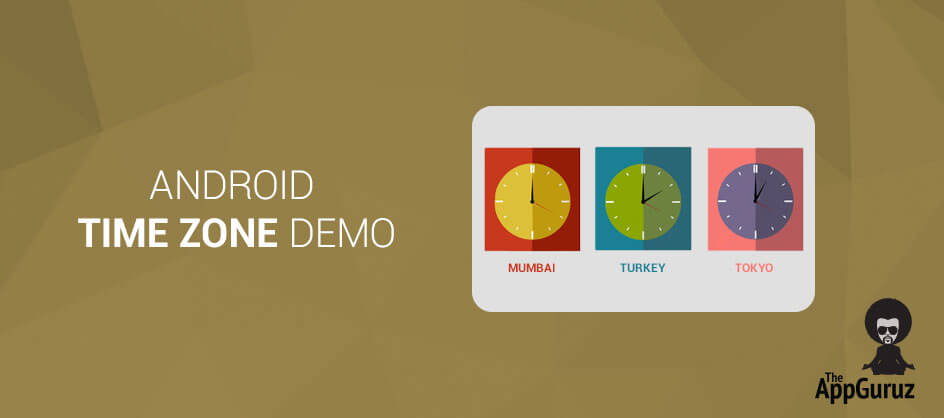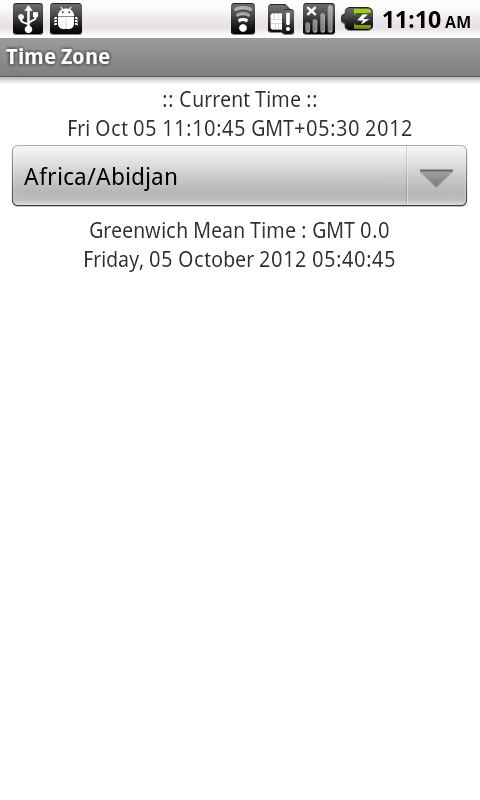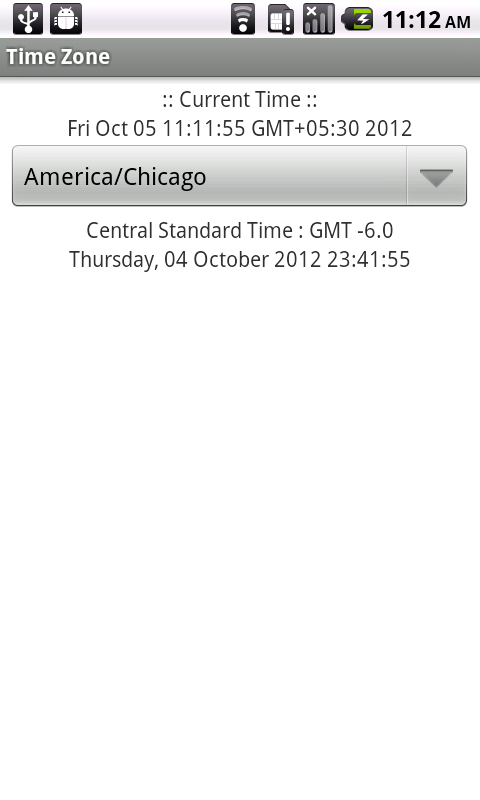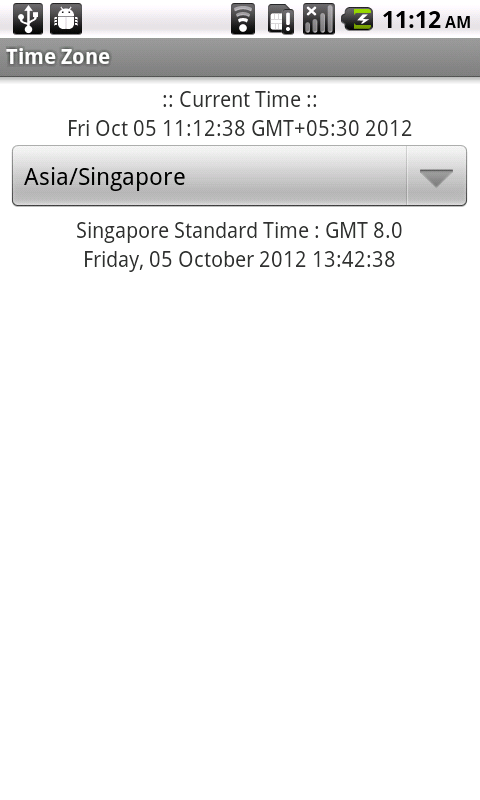## Objective

Main objective of this tutorial is to help you to get Time according to the Different Time Zone.

You will get Final Output:

•••## Step 1 MainActivity.java

First create new Android Project. Add Following into your Main Activity class

``````private Spinner spinnerAvailableID;
private Calendar current;
private TextView textTimeZone, txtCurrentTime, txtTimeZoneTime;
private long miliSeconds;
private SimpleDateFormat sdf;
private Date resultdate;``````

Then in our Main Activity class we need all IDs of the different Time Zone. So we use.

``String[] idArray = TimeZone.getAvailableIDs();``

## Step 2 getGMTTime() method implementation

Add new method for converting the current time into GMT (Greenwich Mean Time).

``````private void getGMTTime() {
current = Calendar.getInstance();
txtCurrentTime.setText("" + current.getTime());

miliSeconds = current.getTimeInMillis();

TimeZone tzCurrent = current.getTimeZone();
int offset = tzCurrent.getRawOffset();
if (tzCurrent.inDaylightTime(new Date())) {
offset = offset + tzCurrent.getDSTSavings();
}

miliSeconds = miliSeconds - offset;

resultdate = new Date(miliSeconds);
System.out.println(sdf.format(resultdate));
}``````

## Step 3 onCreate() Method

``````        spinnerAvailableID = (Spinner) findViewById(R.id.availableID);
textTimeZone = (TextView) findViewById(R.id.timezone);
txtCurrentTime = (TextView) findViewById(R.id.txtCurrentTime);
txtTimeZoneTime = (TextView) findViewById(R.id.txtTimeZoneTime);

sdf = new SimpleDateFormat("EEEE, dd MMMM yyyy HH:mm:ss");

android.R.layout.simple_spinner_item, idArray);

getGMTTime();

spinnerAvailableID
.setOnItemSelectedListener(new OnItemSelectedListener() {

@Override
View view, int position, long id) {
getGMTTime();
String selectedId = (String) (parent
.getItemAtPosition(position));

TimeZone timezone = TimeZone.getTimeZone(selectedId);
String TimeZoneName = timezone.getDisplayName();

int TimeZoneOffset = timezone.getRawOffset()
/ (60 * 1000);

int hrs = TimeZoneOffset / 60;
int mins = TimeZoneOffset % 60;

miliSeconds = miliSeconds + timezone.getRawOffset();

resultdate = new Date(miliSeconds);
System.out.println(sdf.format(resultdate));

textTimeZone.setText(TimeZoneName + " : GMT " + hrs + "."
+ mins);
txtTimeZoneTime.setText("" + sdf.format(resultdate));
miliSeconds = 0;
}

@Override

}
});``````